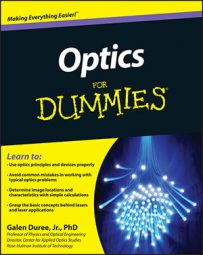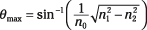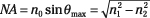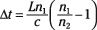##### Optics For DummiesBesides imaging, fiber-optic networks are probably the largest application of optics. Fiber optics are very long, thin glass fibers that transfer information-bearing light from one place to another, but that may not be in direct sight of each other. You need to be aware of a few characteristics of the particular fiber you're using so that you can ensure the information is accurately transmitted from one end of the fiber to the other. The following equations cover three of the basic parameters necessary for proper use of optical fibers.

• The maximum acceptance angle for a fiber: This angle is the largest angle of incidence at which light can enter the end of the fiber and be totally internally reflected inside the fiber. Angles of incidence larger than this angle will transmit through the sides of the fiber and not make it to the other end. The equation for this angle is• The numerical aperture for a fiber: The numerical aperture is a measure of the light-gathering power of the fiber. It has a maximum value of 1 (all the light remains trapped inside the fiber) and a minimum value of 0 (only light incident at an angle of 0 degrees on the end of the fiber remains trapped in the fiber). Use this equation:• Intermodal dispersion in a fiber: This characteristic measures the difference in time that different fiber modes take to reach the end of the fiber. The larger this time difference, the shorter the fiber has to be so that the information on this light doesn't turn into junk. Here's the equation: